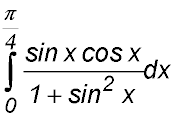Dr. J's Maths.com
Where the techniques of Maths
are explained in simple terms.

Calculus - Integration - Reverse Chain Rule - Multi-function expressions.
Test Yourself 1.

Before you start the serious stuff, read the following to show how logs and exponentials can combine to make you happy!!!!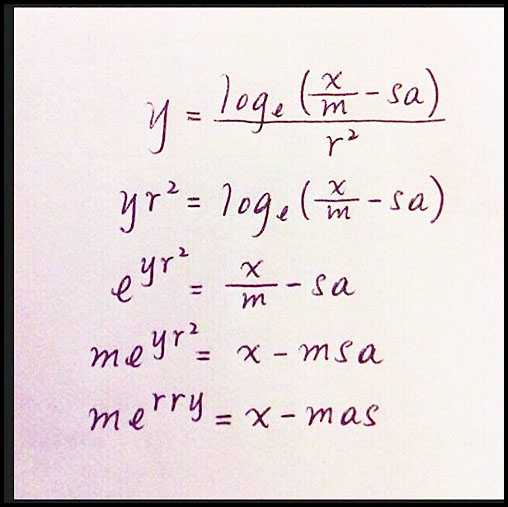Now to the serious stuff:

 Exponential and log functions. 1.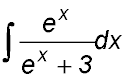. Hint.Let u = ex + 3. 2. (i) Prove that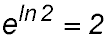. (ii) Hence prove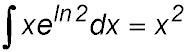3.. 4. Evaluate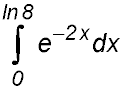Answer.63/128 5. Show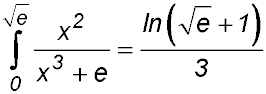. 6.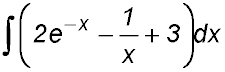7.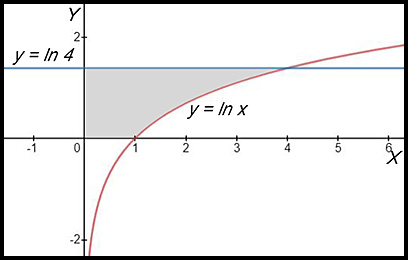The diagram above shows the curve y = loge x and the line y = loge 4. (i) Solve the equation loge x = loge 4. Hence write down the coordinates of the point of intersection of the two functions. (ii) Show that the area of the shaded region is 3 u2. (iii) Find the exact value of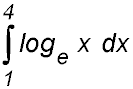. 8.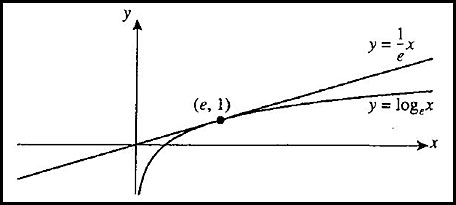The diagram shows the graphs of y = loge x and the tangent to y = loge x at the point (e, 1). (i) Show that the equation of the tangent is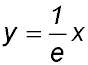. (ii) Explain why for all positive values of x: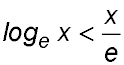except for x = e. (iii) Hence show that. Exponential and trig functions. 9.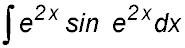Hint.Let u = e2x. 10.. 11.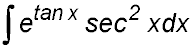Hint.Let u = tan x. 12.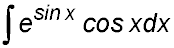Hint.Let u = sin x. 13.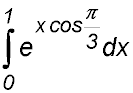and simplify your answer fully. 14.Log and trig functions. 15.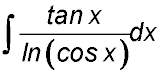Hint.Let u = ln (cos x). 16. (i) Differentiate loge (cosx) with respect to x. (ii) Hence or otherwise show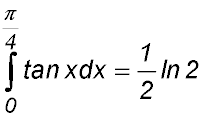17. (i) Sketch the graphs of y = 2 sinx and y = tan x for 0 ≤ x ≤ π/2. Mark A as the point of intersection of the graphs where x ≠ 0. (ii) Find the coordinates of point A. (iii) Show that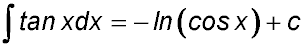(iv) Find the area of the region between the two curves from the origin to Point A. Answer.(i) A is (π/3, √3)(iii) Area = (1 - ln2) u2. 18. Evaluate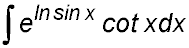. Hint.Let u = ln sin x. 19.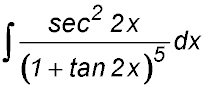20. Evaluate